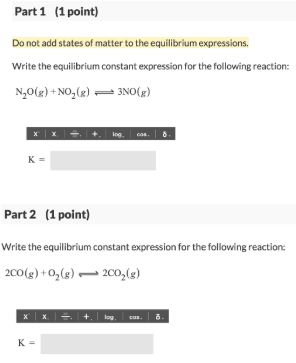# Problem: Part 1 (1 point) Do not add states of matter to the equilibrium expressions.Write the equilibrium constant expression for the following reaction: N2O (g) + NO2 (g) ⇌ 3NO (g) Part 2 (1 point) Do not add states of matter to the equilibrium expressions.Write the equilibrium constant expression for the following reaction: 2CO (g) + O2 (g) ⇌ 2CO2 (g)

###### FREE Expert Solution
90% (218 ratings)###### Problem Details

Part 1 (1 point)

Do not add states of matter to the equilibrium expressions.

Write the equilibrium constant expression for the following reaction:

N2O (g) + NO2 (g) ⇌ 3NO (g)

Part 2 (1 point)

Do not add states of matter to the equilibrium expressions.

Write the equilibrium constant expression for the following reaction:

2CO (g) + O2 (g) ⇌ 2CO2 (g)